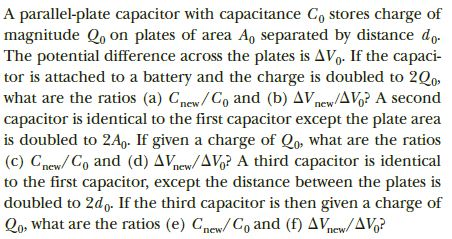# A parallel-plate capacitor with capacitance C, stores charge ofmagnitude Q, on plates of area A, separated by distance do.The potential difference across the plates is AVg. If the capaci-tor is attached to a battery and the charge is doubled to 2Q0what are the ratios (a) Cmew/Co and (b) AVnew/AVo? A secondcapacitor is identical to the first capacitor except the plate areais doubled to 2A,- If given a charge of Qo, what are the ratios(c) Cnew/ Co and (d) AVnew/AVg? A third capacitor is identicalto the first capacitor, except the distance between the plates isdoubled to 2d,. If the third capacitor is then given a charge ofQo, what are the ratios (e) Cnew/C, and (f) AVnew/AVg?пеwпеwпew

Question
14 viewshelp_outlineImage TranscriptioncloseA parallel-plate capacitor with capacitance C, stores charge of magnitude Q, on plates of area A, separated by distance do. The potential difference across the plates is AVg. If the capaci- tor is attached to a battery and the charge is doubled to 2Q0 what are the ratios (a) Cmew/Co and (b) AVnew/AVo? A second capacitor is identical to the first capacitor except the plate area is doubled to 2A,- If given a charge of Qo, what are the ratios (c) Cnew/ Co and (d) AVnew/AVg? A third capacitor is identical to the first capacitor, except the distance between the plates is doubled to 2d,. If the third capacitor is then given a charge of Qo, what are the ratios (e) Cnew/C, and (f) AVnew/AVg? пеw пеw пew fullscreen
check_circle

Step 1

Case 1: When the charge is doubled,

Write the expression for capacitance of a parallel plate capacitor

Step 2

(a) Capacitance doesn’t depend on the charge stored and hence doesn’t change by increasing the charge stored. Thus,

Step 3

(b) write the expression for potential differ...

### Want to see the full answer?

See Solution

#### Want to see this answer and more?

Solutions are written by subject experts who are available 24/7. Questions are typically answered within 1 hour.*

See Solution
*Response times may vary by subject and question.
Tagged in

### Electrostatic Potential and Capacitance